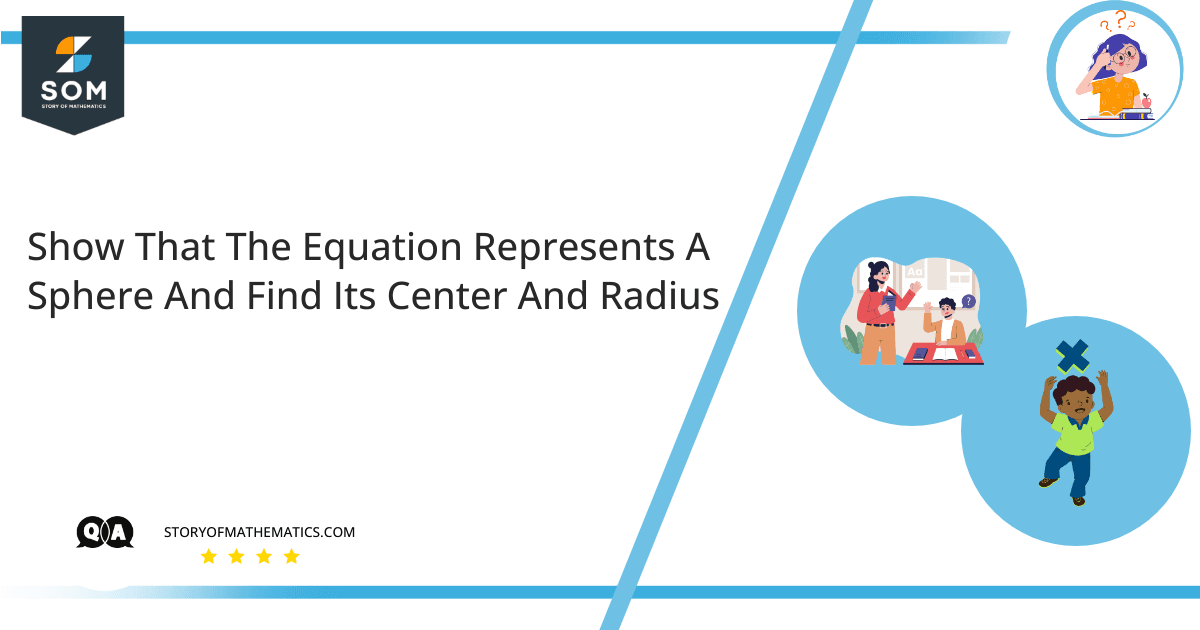# Show that the equation represents a sphere and find its center and radius.

• $x^2+y^2+z^2+8x-6y+2z+17=0$

The main objective of this question is to prove that the given equation is for a sphere and also to find the center and radius for a given sphere equation.This question uses the concept of the sphere. A sphere is a round, three-dimensional object like a ball or moon where each point on its surface has an equal distance from its center. One of the properties of the sphere is that it is perfectly symmetrical and it’s not polyhedron. The other property of the sphere is its mean curvature, and circumference and width are constant.

The given equation is:

$=x^2+y^2+z^2+8x-6y+2z+17=0$

We have to prove, that it is a sphere equation and finds the center and radius of the given sphere equation.

Imagine a sphere with its centre $C(h,j,l)$ and its radius $r$.

We have formula for sphere as:

$=(x-h)^2 + (y-k)^2 +(z-l)^2 = r^2(l)$

where $(h,k,l)$ is the sphere center and its radius is represented by $r$.

Rearranging the given equation results in:

$(x^2 +8x +4^2 -4^2)+(y^2-6y+3^2-3^2)+(z^2+2z-1^2-1^2)+17=0$

$(x^2 +8x +4^2 )+(y^2-6y+3^2)+(z^2+2z-1^{20})+(-16-9-1)+17=0$

$(x^2 +8x +4^2 )+(y^2-6y+3^2)+(z^2+2z-1^{20})+(-16-10)+17=0$

$(x^2 +8x +4^2 )+(y^2-6y+3^2)+(z^2+2z-1^{20})+(-26)+17=0$

$(x^2 +8x +4^2 )+(y^2-6y+3^2)+(z^2+2z-1^{20})-26+17=0$

Moving $-26$ to the right side results in:

$(x^2 +8x +4^2 )+(y^2-6y+3^2)+(z^2+2z-1^{20})+17=26$

By shifting $17$ to the right side results in:

$(x^2 +8x +4^2 )+(y^2-6y+3^2)+(z^2+2z-1^{20})+=26-17$

Subtracting the right side term results in:

$(x^2 +8x +4^2 )+(y^2-6y+3^2)+(z^2+2z-1^{20})=9$

$[x-(-4)]^2 +(y-3)^2 +[z-(-1)]^2=(3)^2(2)$

Now comparing the two-equations, we get:

$h$=-4.

$k$=3.

$l$=-1.

$r$=3.

Therefore, the sphere center is $(-4,3,1)$  and its radius is $3$.

For the given sphere equation, it is proved that it’s of the sphere and the center is $(-4,3,1)$, with a radius of $3$.

## Example

Show that the given two equations are for the sphere and also find the center and radius for these two-sphere equations.

$2x^2+2y^2+2z^2=8x-24z+1$

$x^2+y^2+z^2-2x-4y+8z=15$

Imagine a sphere with its centre $C(h,j,l)$ and its radius $r$. It is represented by formula as:

$=(x-h)^2 + (y-k)^2 +(z-l)^2 = r^2(l)$

where $(h,k,l)$ is the sphere center and its radius is represented by $r$.

The given sphere equation is:

$2x^2+2y^2+2z^2=8x-24z+1$

Dividing the given equation by $2$ results in:

$x^2-4x+y^2+z^2+12z=\frac{1}{2}$

For a complete square, we have to add 40 to both sides.

$x^2-4x+4+y^2+z^2+12z+36=\frac{1}{2} + 40$

Adding 40 to both sides result in:

$x^2-4x+y^2+z^2+12z+40=\frac{81}{2}$

Make a square term so that we can compare it with the equation of a sphere.

$(x-2)^2 +y^2 +(z+6)^2=\frac{81}{2}$

Now for the $2^{nd}$, given equation, we have to prove its sphere equation and also to find the center and radius of this equation.

$(x^2+2x)+(y^2+4y)+(z^2+8z)=15$

By simplifying the given equation,we get:

$(x-1)^2 +(y-2)^2 +(z-(-4)^2)=6^2$

Now, this equation is in the form of a standard sphere equation. By comparing this equation with the standard sphere equation results in:

$center=(1,2,-4)$

$radius=6$

Hence, it is proved that the given equation is for sphere with center $(2,0,-6)$ and radius $\frac{9}{\sqrt{2}}$ and for the $2^{nd}$ equation   $x^2+y^2+z^2-2x-4y+8z=15$  is also for sphere and its center is   $(1,2,-4)$ and radius is $6$.